Next: Line Integrals Up: Vectors Previous: The Vector Product

## Vector Calculus

Suppose that vector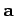varies with time, so that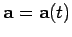. The time derivative of the vector is defined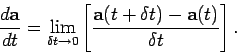(38)

When written out in component form this becomes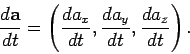(39)

Suppose thatis, in fact, the product of a scalar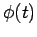and another vector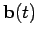. What now is the time derivative of? We have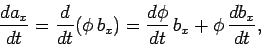(40)

which implies that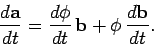(41)

It is easily demonstrated that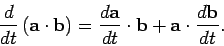(42)

Likewise,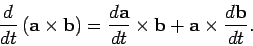(43)

It can be seen that the laws of vector differentiation are fairly analogous to those in conventional calculus.Next: Line Integrals Up: Vectors Previous: The Vector Product
Richard Fitzpatrick 2007-07-14